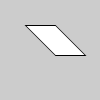# shear_x()#

Shears a shape around the x-axis the amount specified by the `angle` parameter.

## Examples#```def setup():
py5.translate(py5.width/4, py5.height/4)
py5.shear_x(py5.PI/4.0)
py5.rect(0, 0, 30, 30)
```

## Description#

Shears a shape around the x-axis the amount specified by the `angle` parameter. Angles should be specified in radians (values from `0` to `TWO_PI`) or converted to radians with the radians() function. Objects are always sheared around their relative position to the origin and positive numbers shear objects in a clockwise direction. Transformations apply to everything that happens after and subsequent calls to the function accumulates the effect. For example, calling `shear_x(PI/2)` and then `shear_x(PI/2)` is the same as `shear_x(PI)`. If `shear_x()` is called within the `draw()`, the transformation is reset when the loop begins again.

Technically, `shear_x()` multiplies the current transformation matrix by a rotation matrix. This function can be further controlled by the push_matrix() and pop_matrix() functions.

Underlying Processing method: shearX

## Signatures#

```shear_x(
angle: float,  # angle of shear specified in radians
/,
) -> None
```

Updated on September 01, 2022 16:36:02pm UTC